AVR PAL generation
Short description of hobbyist project

"...Imagination is more important than knowledge.
Knowledge is limited. Imagination encircles the world..."
Albert EinsteinPAL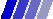PAL (Phase Alternation by Line) was developed to fix NTSC (National Television System Committee) problems with color. Each PAL frame consist of 625 lines, 25 frames per second. All image data is in scan-lines.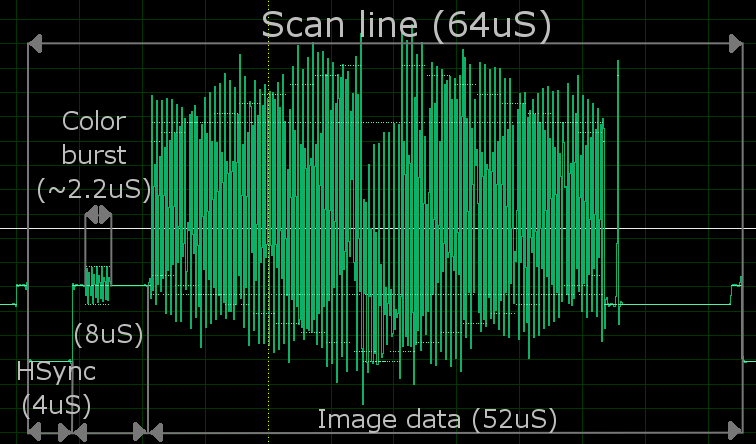Pic 1. Normal scanline.

There is 625 scanlines. Not all of them are visible - some are used by vertical retrace and teletext. More information about it you can find here. Scanlines during vertical retrace are different than normal ones, the 0V pulses arrives twice as fast as in normal scanlines.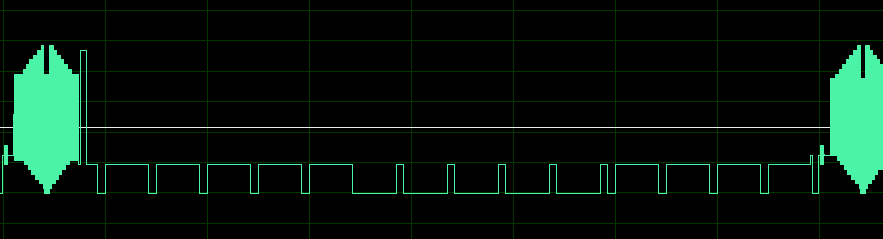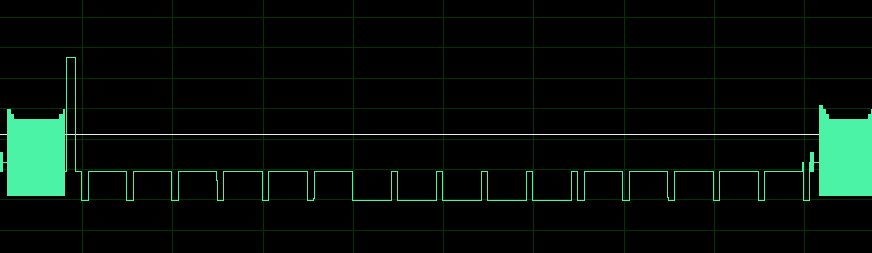Pic 2. Vertical retraces.

The color generation is described in next section.

PAL standard defines also how sound should be transmitted, but composite-video contains no audio signal. We are transmitting Video signal, but we can also try to transmit teletext information.

The ColorColor in PAL is coded using YUV colorspace model, where Y stands for luminance (brightness), and U V stands for chrominance. This is not the usual way that we used to represent color information (like RGB or CMYK models). The fact that we are using this method is mainly because the inventors of PAL had to made it compatible with older black&white television systems.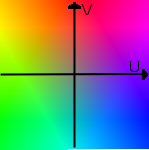Pic 3. YUV colorspace. [ Y ]   [ 0.299  0.587  0.114 ] [ R ] [ U ] = [-0.147 -0.289  0.436 ]*[ G ] [ V ]   [ 0.615 -0.515 -0.100 ] [ B ] [ R ]   [ 1  0      1.14  ] [ Y ] [ G ] = [ 1 -0.394 -0.581 ]*[ U ] [ B ]   [ 1  2.028  0     ] [ V ] Pic 4. RGB to YUV conversions.

PAL is using quadrature modulation with phase shift for color. The Y phase is changing every line by 180 deg.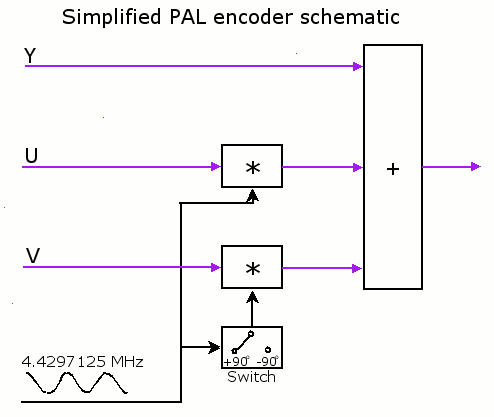Pic 5. PAL Encoder.

{ S(t) = Y + U cos (wt) + V sin (wt)   for even lines
{ S(t) = Y + U cos (wt) - V sin (wt)   for odd lines
{ w=2*pi*f, f= 4.4297125 MHz

In fact, there is no possibility for video-decoder to discover the phase of signal. This information is sent every line during color-burst phase, when transmitter sends the sinus signal with correct phase. That allows receiver to synchronise phase every line.

MathematicsMost projects with microcontroler PAL generation are based on equation:

a sin(x) + b cos(x) = A sin (x + B),
A= sqrt( a^2 + b^2 ), tg(B) = a/b

 Simply: why we have to calculate sinus and cosinus functions for each sample when we can only calculate one sinus with phase shift?

I decided to go some other way. Since my microcontroler is running four times as fast as the color signal, I simply write four equations for each sample of signal:

S0 = Y + U cos(2pi*0/4) +/- V sin (2pi*0/4)
S1 = Y + U cos(2pi*1/4) +/- V sin (2pi*1/4)
S2 = Y + U cos(2pi*2/4) +/- V sin (2pi*2/4)
S3 = Y + U cos(2pi*3/4) +/- V sin (2pi*3/4)

In fact, the values of cos and sin are well known for this angles so we get...

S0 = Y + U
S1 = Y +/- V
S2 = Y - U
S3 = Y -/+ V

...what is very easy to calculate, even real-time.

 Simply: why we have to calculate sinus function for each sample when we can only add or subtract values?

But there is still one problem with this solution - when I generating color signal I don't have time to do anything else. That's bad. We cannot make loops and other things. So I tried to make color with 2 samples only, but without changing color-burst. First I tested it in device and it works, so I went back to paper to find out what am I really doing ... ;) To solve this problem we have to know that PAL color signal is same for two horizontal lines - odd and even pair. The decoder is applying addition operation to them to get the U, and substraction to get the V. So in fact we at start got 4 samples:

(S00, S01) at first line
(S10, S11) at second line

when we add and subtract them we got pairs:

U: (S00+S10, S01+S11)
V: (S00-S10, S01-S11)

So, basically we are modulating U and V by using 2 samples for each. In fact, the thing that is important is fact that we can control the amplitude of them, which is:

U = S01+S11 - (S00+S11)
V = S01-S11 - (S00-S10) = S01+S10 - (S00+S11)

 Simply: why we have to modulate 8 samples to get the color for two pixels when we can only use 4 samples and have free time then?

Equations are simplified to illustrate the idea.

Pic 4. Simulation of PAL color coding.

 Topics: 1.Ideas 2.PAL 3.Problems

From TV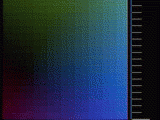2-sample plasma effect on TV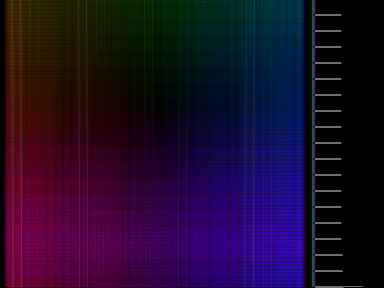4-sample plasma effect on TV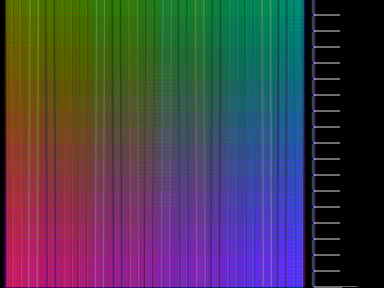4-sample plasma effect on TV

Devboard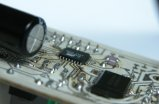Dev-board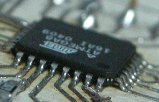Devboard - ATMEL zoomed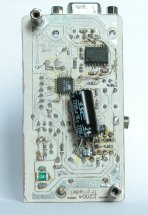Devboard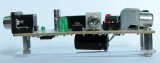Devboard - status LED,
microphone and reset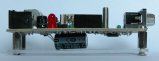Devboard - power LED,
programer connector and
joystick connector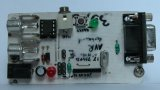Devboard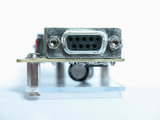Devboard - RS232 port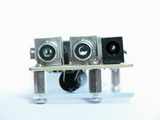Devboard - video&audio out,
power in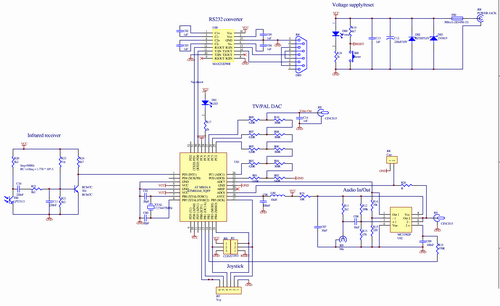Devboard - schematics,

 Download: Plasma.zip (30kB) Plasma_2s.zip (30kB) Plasma.wav (1.7MB)

 Links: AVR PAL color bar SX/PIC Tetris AVR GCC AVR Studio ATmega8

 Contact me? My homepage Mail me

 Visitor nr: